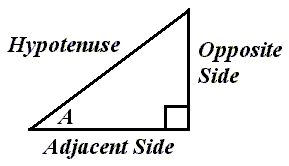# The Right Triangle and the Sine FunctionRelative to angle A, this is how the sides of a right triangle would be labeled.

The sine of angle A equals the length of the opposite side divided by the length of the hypotenuse.

We could write:

sin(A) = opp / hyp

So, for example, if the length of the opposite side was 6 and the length of the hypotenuse was 10, then we would write:

sin(A) = 6 / 10

sin(A) = 0.6000

Custom Search